# Inter Maths Solutions for exercise 6(e) Trigonometric Ratios upto Transformations

Inter Maths 1A solutions for  Trigonometric Ratios upto Transformations exercise 6(e) are given.

These solutions are very easy to understand. Please study the text book lesson very well.

Observe the example problems and solutions. Try them. Observe the given solutions and try them in your own method.

You can see the solutions for Maths 1A text book

You can see the text book solutions for

Inter Maths 1A textbook solutions

Inter Maths 1B textbook solutions

Inter Maths IIA textbook solutions

Inter Maths IIB textbook solutions

Trigonometric Ratios upto Transformations

Exercise 6(a)

Exercise 6((b)

Exercise 6(c)

Exercise 6(d)

Exercise 6(e)

Exercise 6(f)

M

## Inter Maths Solutions for Trigonometric Ratios upto Transformations exercise 6(e)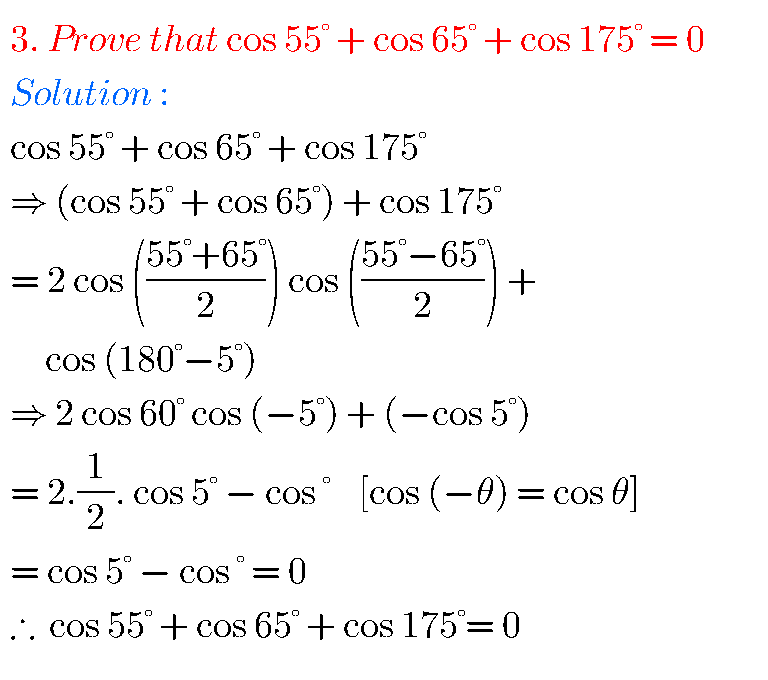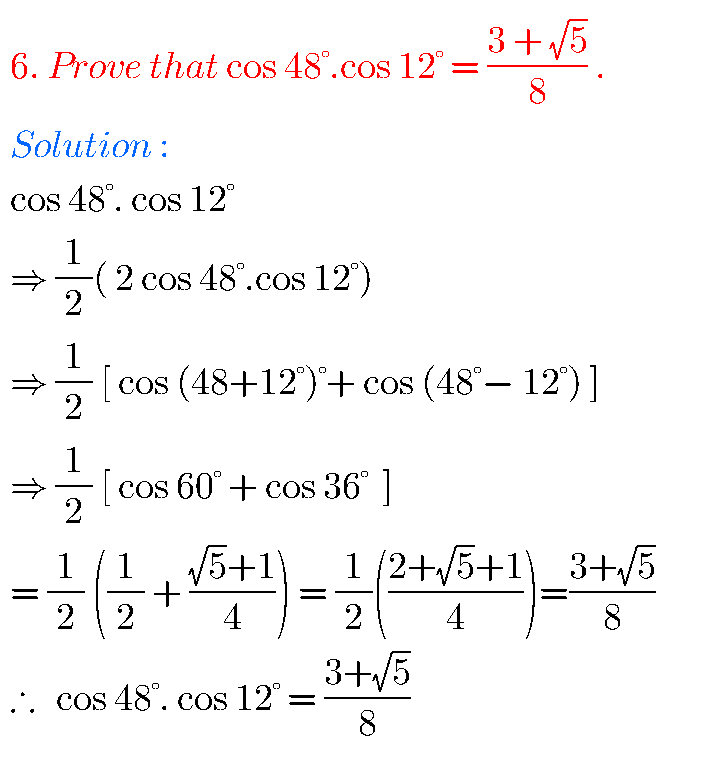## Trigonometric Ratios upto Transformations exercise 6(e)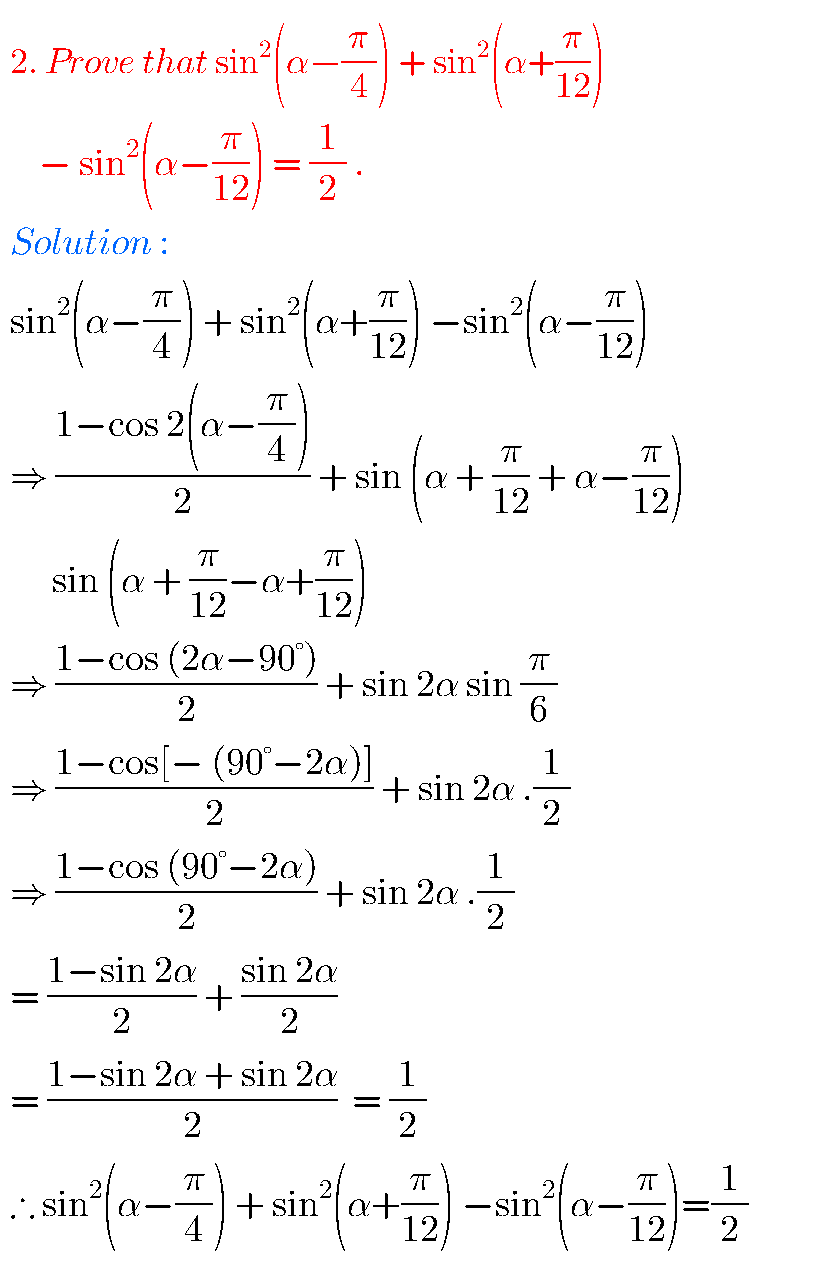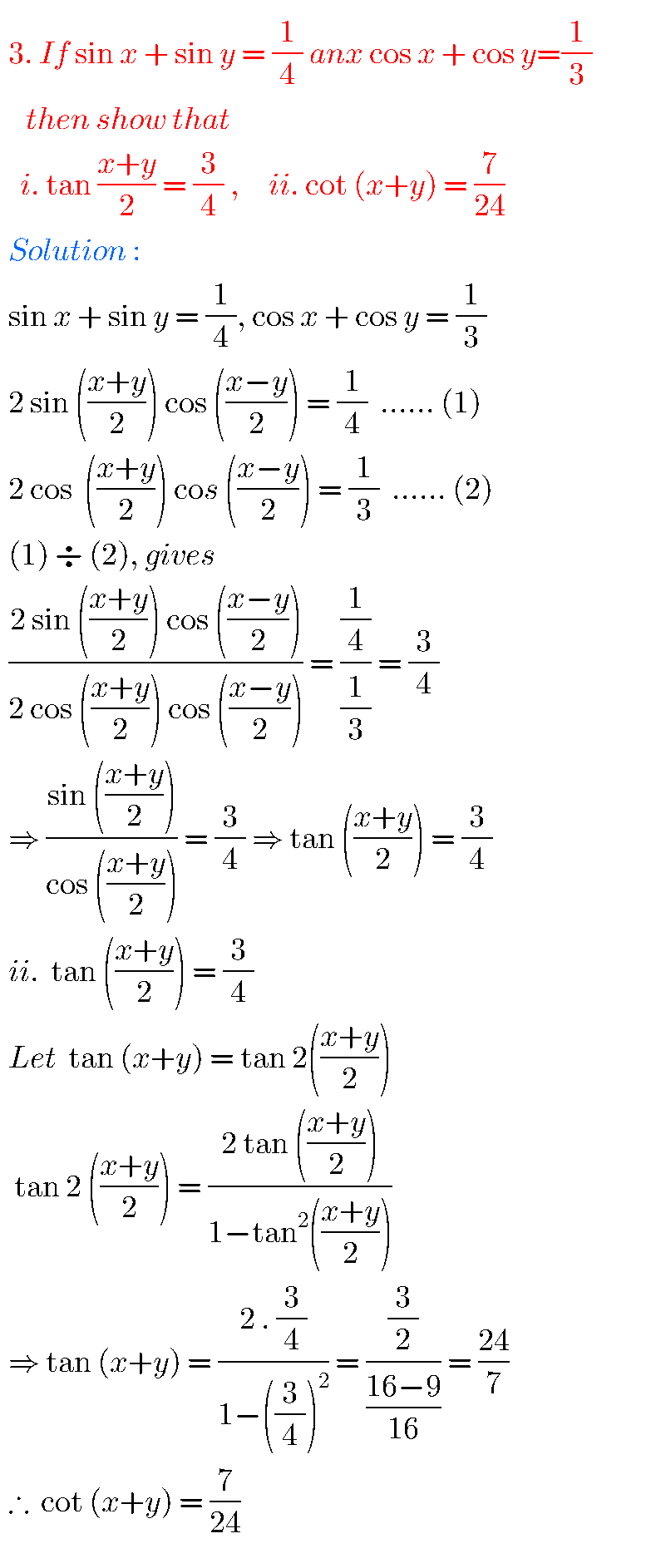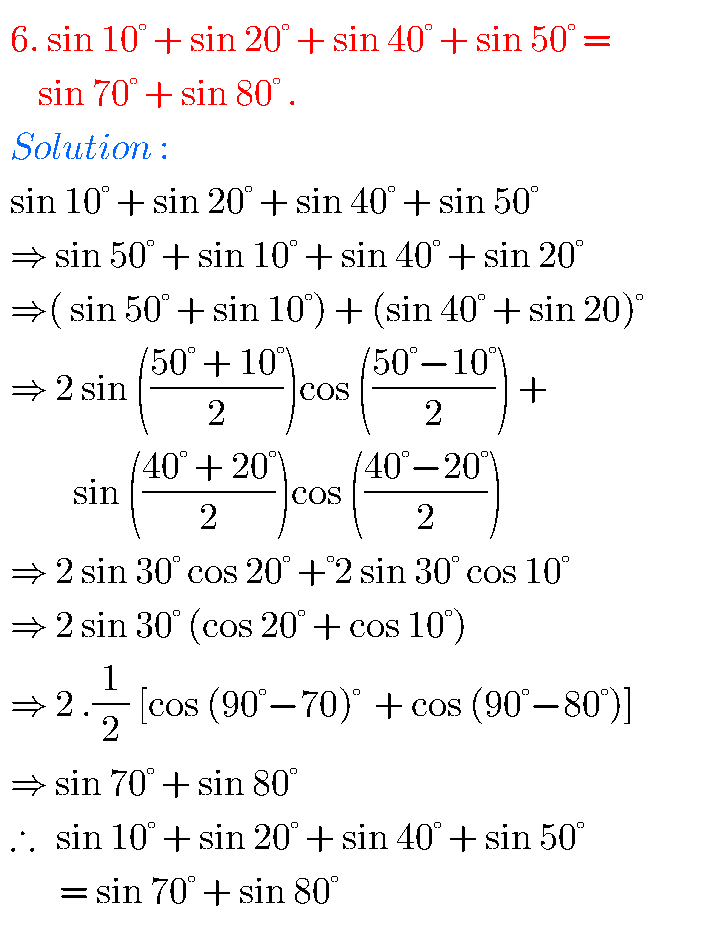## Solutions Inter Maths 1A Solutions for Exercise 6(e)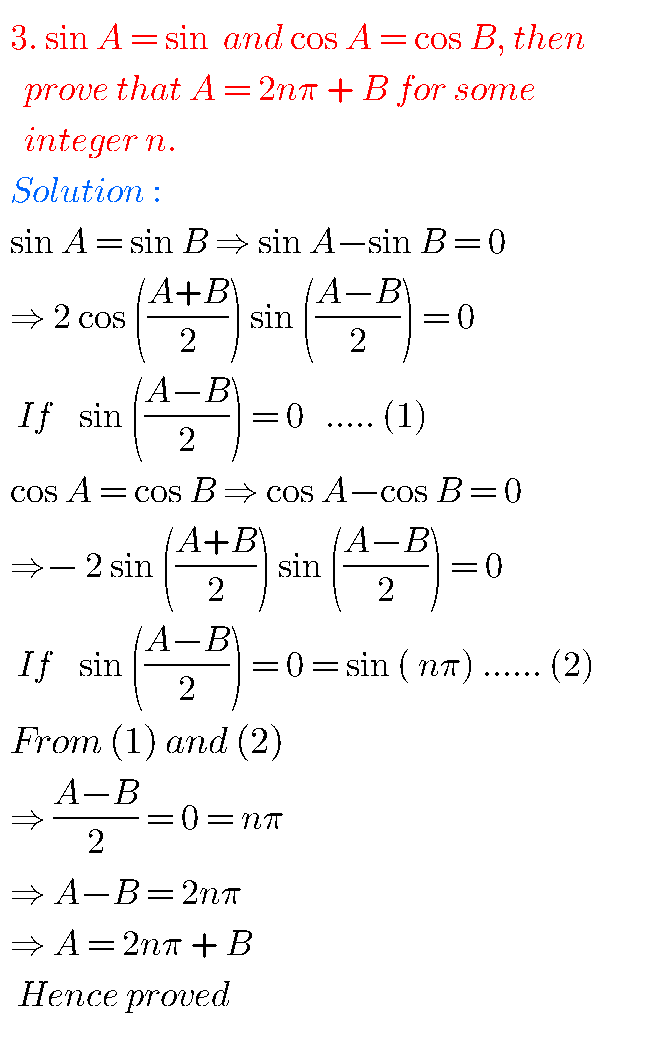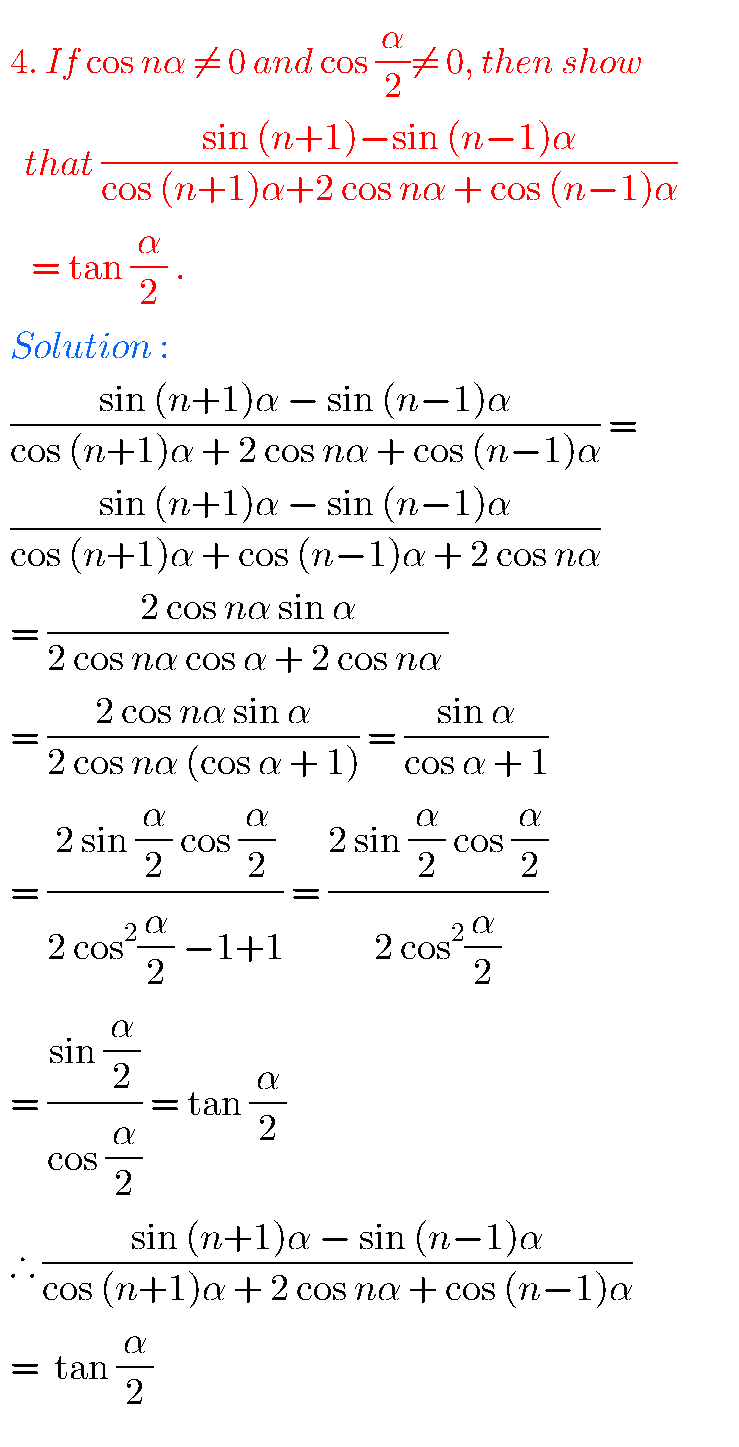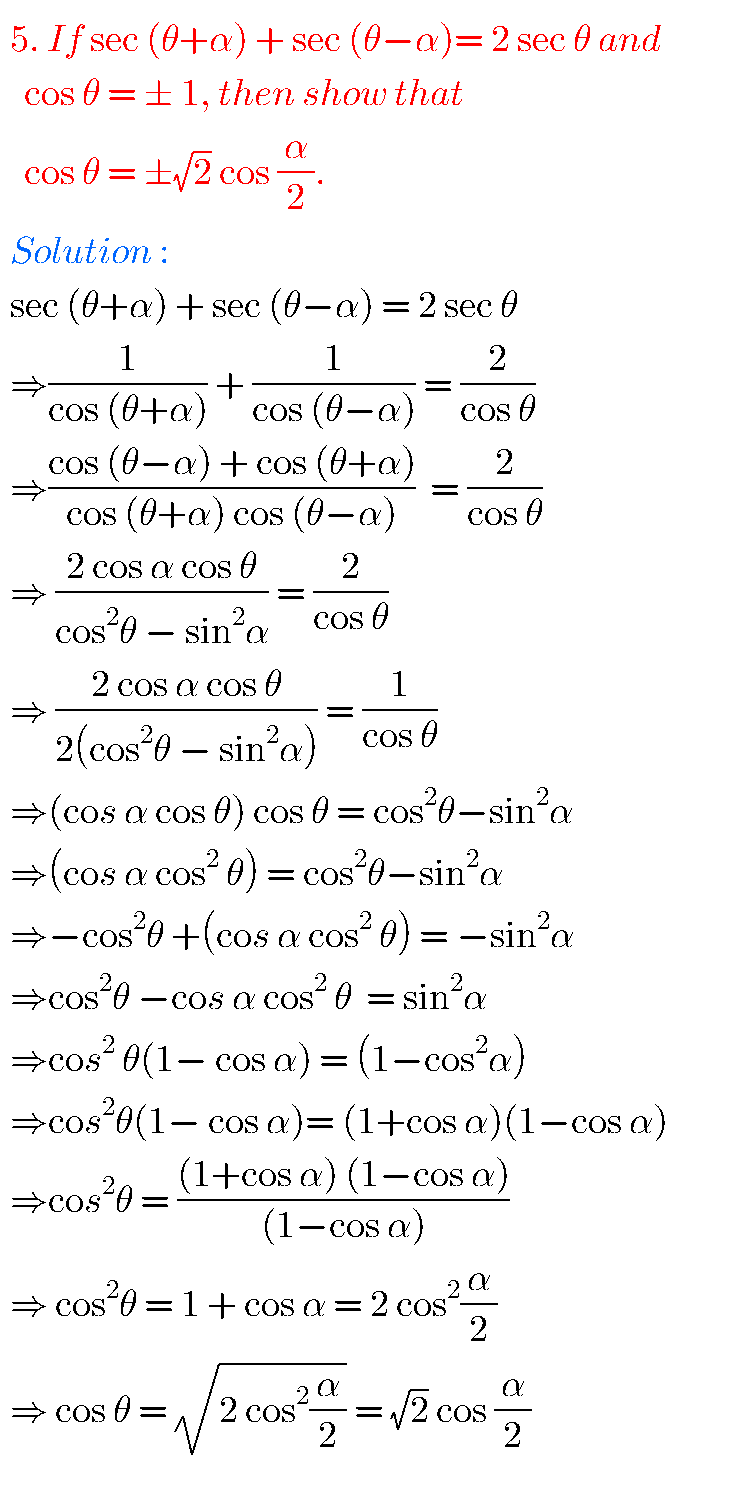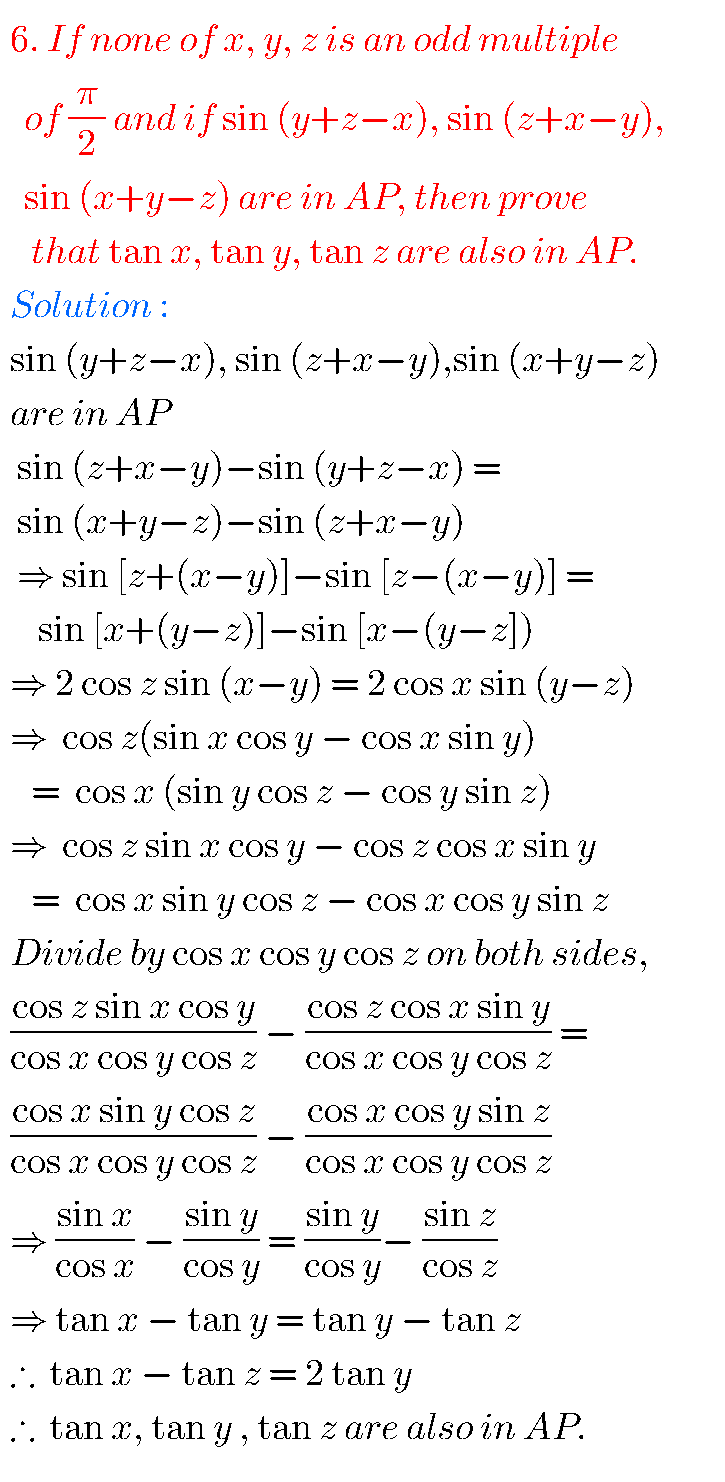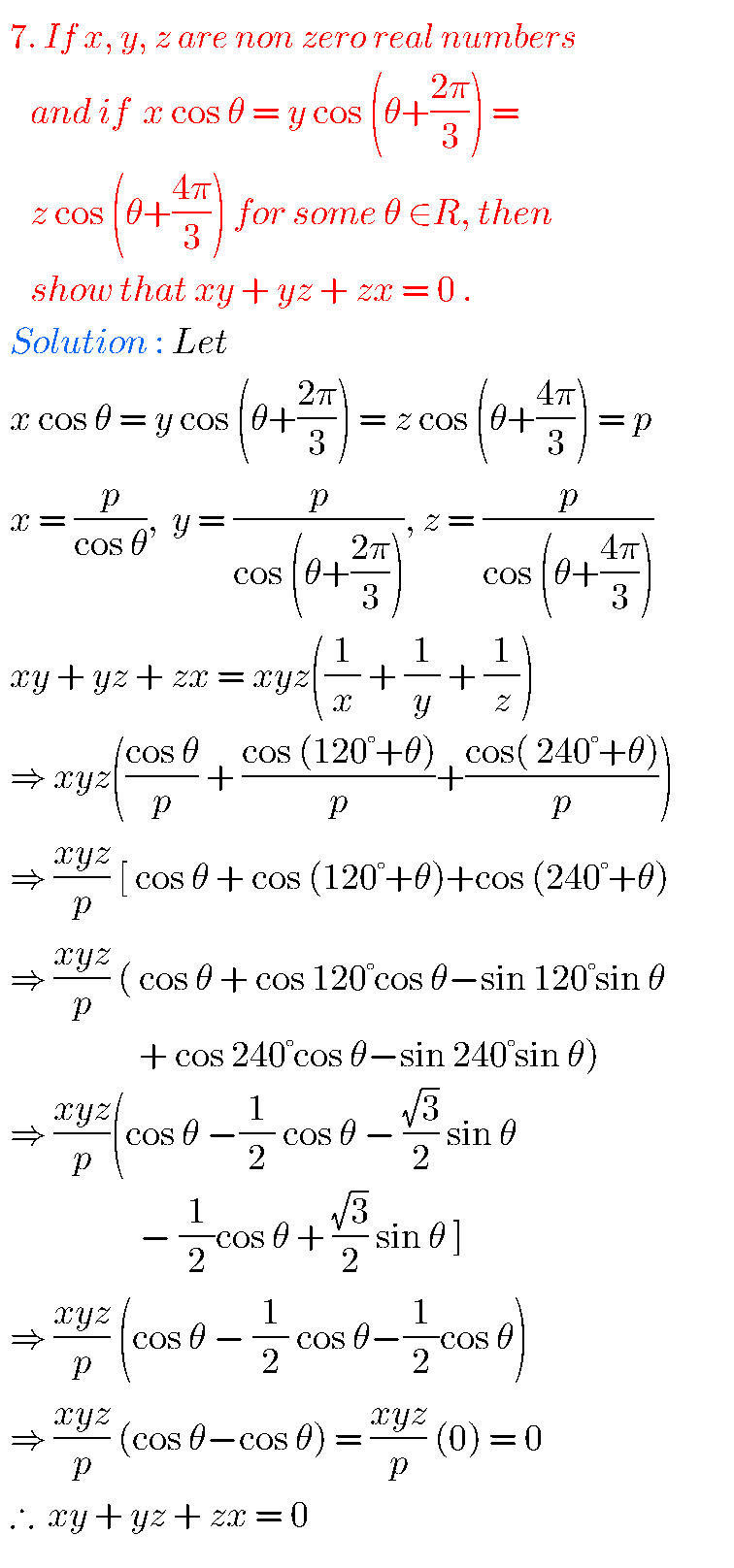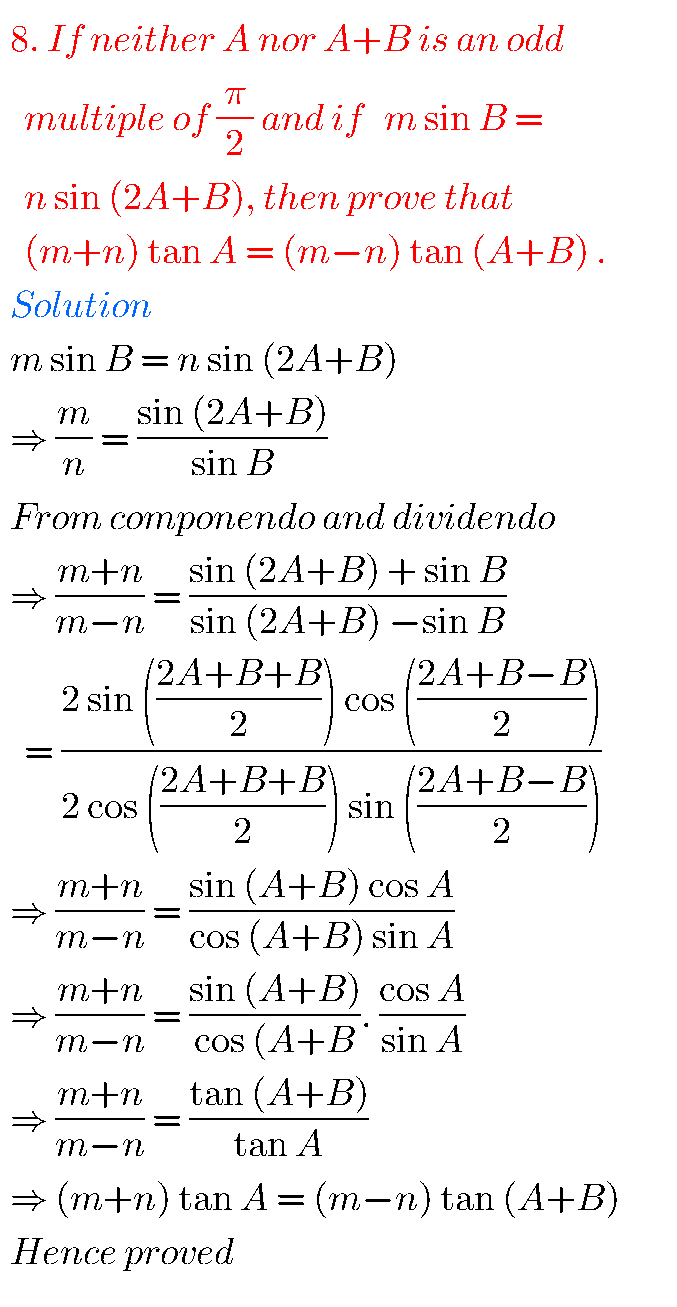Note : Observe the solutions and try them in your own method.

Some other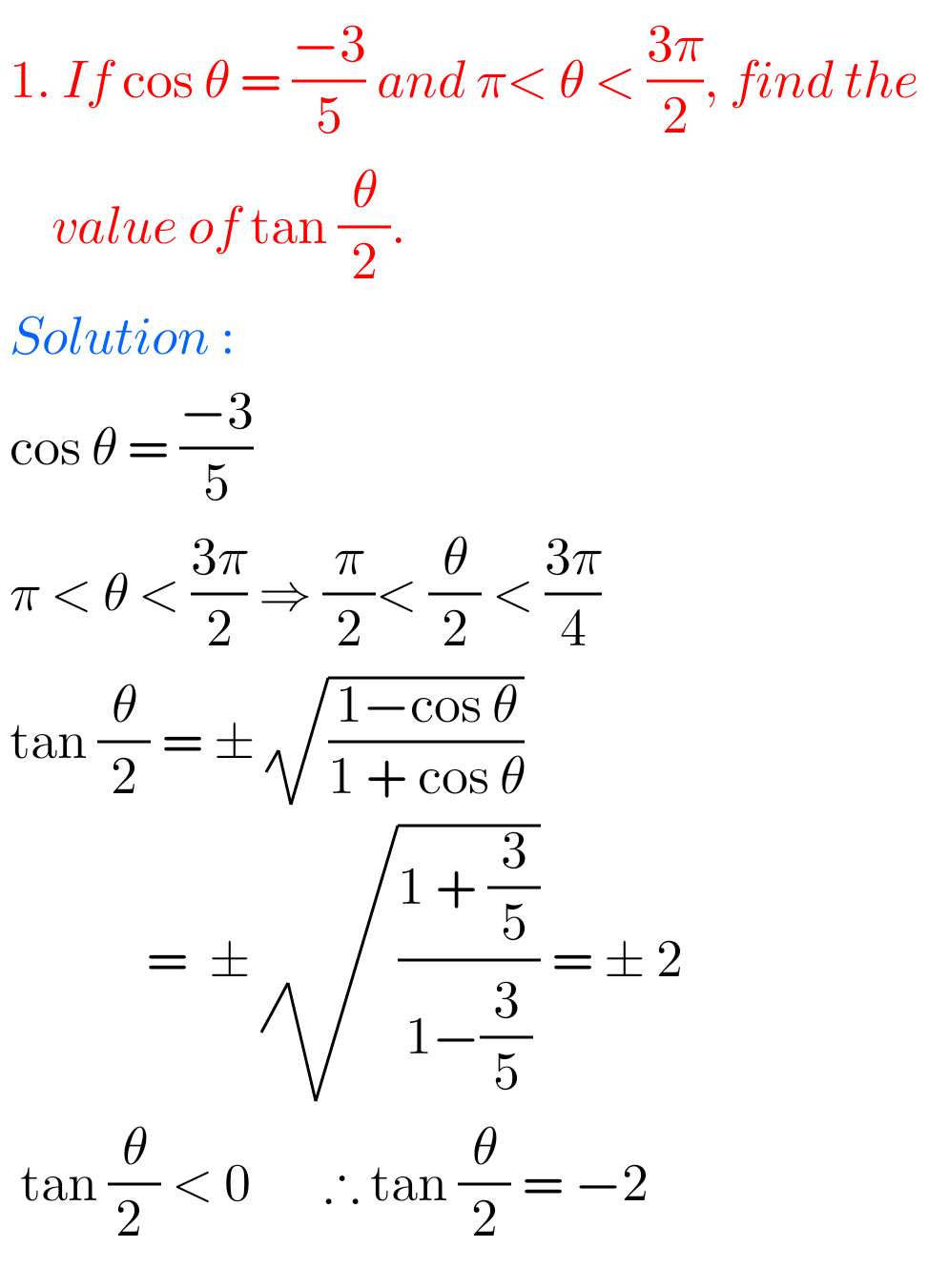You can see the solutions for Inter maths 1A for examination purpose

3. Matrices

Trigonometric ratios upto transformations 2

SSC Maths Solutions

Ncert solutions for Principle of Mathematical Induction

Ncert solutions for Linear Equations in two variables class 8

Ncert solutions for Simple Equations class 7

Ncert maths class 6 chapter 14 solutions

Nios maths 311 book 1 Sets 1.1 solutions